Electricity and Magnetism

# Circuit Behavior - Problem Solving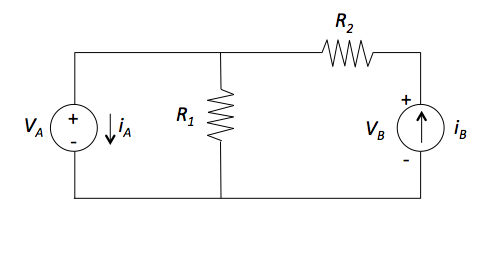Consider the circuit shown in figure above. Suppose that $i_A = 11 \text{ A},$ $i_B = 12 \text{ A},$ $V_A = 20 \text{ V}$ and $V_B = 80 \text{ V}.$ Determine the sum of resistances $R_1$ and $R_2.$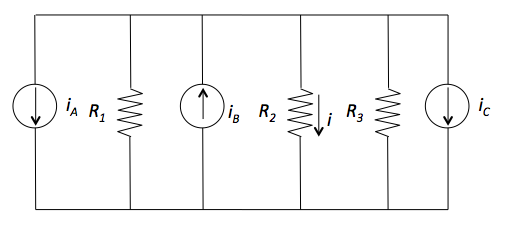Determine the value of the current $i$ in the circuit shown in figure above. Suppose that $i_A= 4 \text{ A},$ $i_B = 1 \text{ A},$ $i_C = 3 \text{ A},$ $R_1 = 6 \ \Omega,$ $R_2 = 2 \ \Omega,$ and $R_3 = 4 \ \Omega.$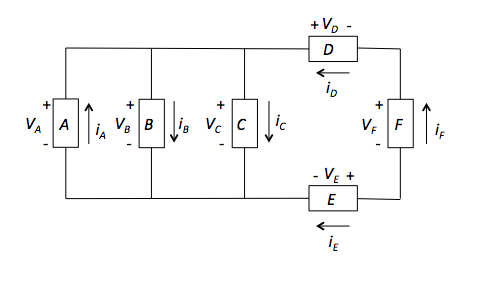Consider the circuit shown in the above figure. The voltages across branches are given by $V_A = 13 \text{ V},$ $V_C = 13 \text{ V},$ $V_D = 3 \text{ V},$ $V_E = -6 \text{ V},$ and $V_F = 16 \text{ V}.$ The currents flowing through branches are given by $i_ A = 12 \text{ A},$ $i_C = 24 \text{ A},$ $i_D = 6 \text{ A},$ $i_E = -6 \text{ A},$ and $i_F = 6 \text{ A}.$ Find the values of the power supplied by branch $B.$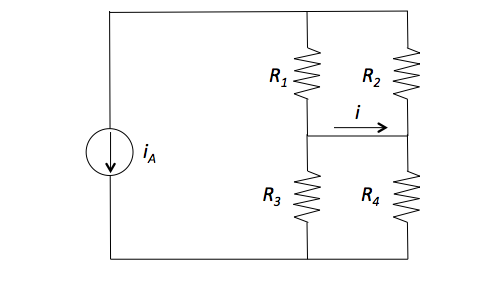Determine the current $i$ in the circuit shown in figure above. Suppose that $i_A = 24 \text{ A},$ $R_1 = 8 \ \Omega,$ $R_2 = 4 \ \Omega,$ $R_3 = 7 \ \Omega,$ and $R_4 = 7 \ \Omega.$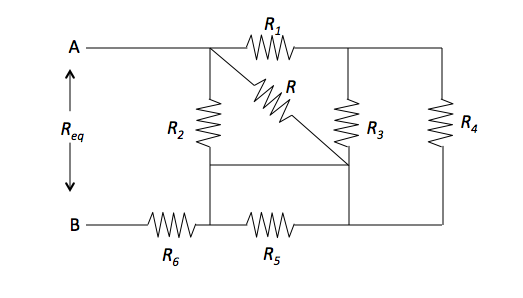Determine the value of the resistance $R$ in the circuit shown in the figure above, given $R_{eq} = 6 \ \Omega,$ $R_1 = 4 \ \Omega,$ $R_2 = 30 \ \Omega,$ $R_3 = 24 \ \Omega,$ $R_4 = 8 \ \Omega,$ $R_5 = 3 \ \Omega,$ and $R_6 = 1 \ \Omega.$

×